RS Aggarwal Class 10 Solutions Chapter 3 - Linear Equations In Two Variables - Ex 3B(3.2)

RS Aggarwal Class 10 Chapter 3 - Linear Equations In Two Variables - Ex 3B(3.2) Solutions Free PDF

The RS Aggarwal Class 10 exercise solutions are considered as an extremely helpful resource for exam preparation. All the questions are prepared in accordance with CBSE, so there is a higher chance of appearing in Class 10 maths question paper. Solving the RS Aggarwal Class 10 solutions for Chapter 3 will not only strengthen their foundation but also give them the confidence to tackle different types of questions. These exercise questions help them in exams as well as doing homework.

The solutions for exercise 3B (3.2) included here are easy to understand, in each step is described in a simple way for a better understanding of the students.  Students of Class 10 should refer to the RS Aggarwal Maths solutions for Chapter 3 while solving the exercise questions from the reference textbook.

Download PDF of RS Aggarwal Class 10 Solutions Chapter 3– Linear Equations In Two Variables – Ex 3B(3.2)

Question 1: On a graph paper, draw a horizontal line X’OX and a vertical line YoY’ as the x-axis and the y-axis respectively.

2x – 3y – 17 = 0, 4x + y – 13 =0

Solution:

Given equations are 2x – 3y – 17 = 0 and 4x + y – 13 =0

Graph of 2x – 3y – 17 = 0:

2x – 3y – 17 = 0 $\Rightarrow y = \frac{2x – 17}{3}$

Putting x = 1, we get y = -5

Putting x = 4, we get y = -3

Putting x = 7, we get y = -1

Hence, the table is,

x147y-5-3-1

Plot the points A(1, -5), B(4, -3) and C(7, -1) on the graph paper. Join AB and BC to get the graph line AC. Extend it both ways.

Thus, line AC is the graph of 2x – 3y – 17 = 0.

Graph of 4x + y – 13 =0 :

4x + y – 13 =0 $\Rightarrow y = -4x + 13$

Putting x = 4, we get y = -3

Putting x = 2, we get y = 5

Putting x = 3, we get y = 1

Hence, the table is,

x423y-351

Now, on the same graph paper as above plot the points P(2, 5) and Q(3, 1). The point B(4, -3) has already been plotted. Join PQ and QB to get PB. The line PB is the graph of the equation 4x + y – 13 =0 is the solution of the given system of equations.

These graph lines intersect the x-axis at R and S. the region bounded by these lines and the x-axis has been shaded.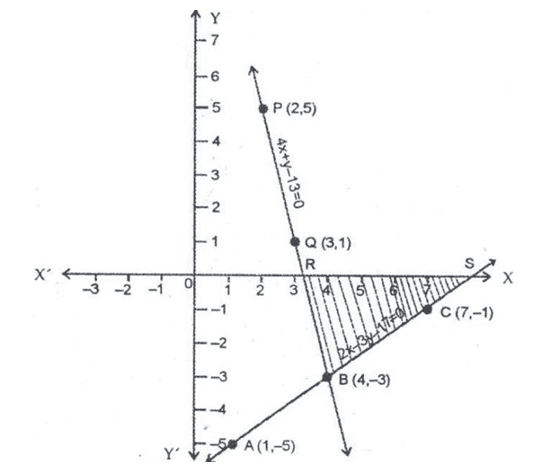Question 2: On a graph paper, draw a horizontal line X’OX and a vertical line YoY’ as the x-axis and the y-axis respectively.

4x – y = 4, 3x + 2y = 14

Solution:

Given equations are 4x – y = 4 and 3x + 2y = 14

Graph of 4x – y = 4:

4x – y = 4 $\Rightarrow y = 4x – 4$

Putting x = 0, we get y = -4

Putting x = 1, we get y = 0

Putting x = 2, we get y = 4

Hence, the table is,

x012y-404

Plot the points A(0, -4), B(1, 0) and C(2, 4) on the graph paper. Join AB and BC to get the graph line AC. Extend it both ways.

Thus, line AC is the graph of 4x – y = 4.

Graph of 3x + 2y = 14 :

3x + 2y = 14  $\Rightarrow y =\frac{14 – 3x}{2}$

Putting x = 0, we get y = 7

Putting x = 2, we get y = 4

Putting x = 4, we get y = 1

Hence, the table is,

x024y741

Now, on the same graph paper as above plot the points P(0, 7) and Q(4, 1). The point C(2, 4) has already been plotted. Join PC and CQ to get PQ.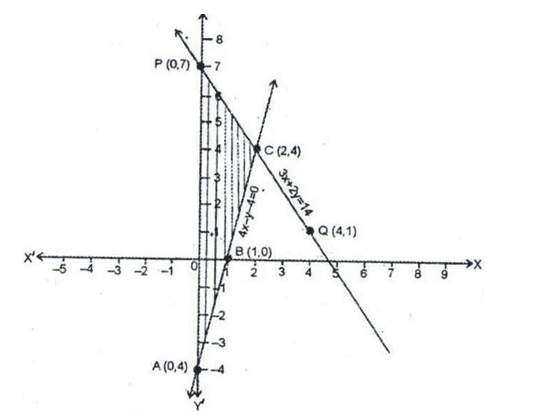The line PQ is the graph of the equation 3x + 2y = 14. The two graph lines intersect at point C(2, 4)

Therefore, x = 2, y = 4 is the solution of the given system of equations. The region bounded by these lines and y-axis has been shown by the shaded area.

Question 3: On a graph paper, draw a horizontal line X’OX and a vertical line YoY’ as the x-axis and the y-axis respectively.

2x – y = 1, x – y = -1

Solution:

Given equations are 2x – y = 1 and x – y = -1

Graph of 2x – y = 1:

2x – y = 1 $\Rightarrow y = 2x – 1$

Putting x = 1, we get y = 1

Putting x = 2, we get y = 3

Putting x = 0, we get y = -1

Hence, the table is,

x120y13-1

Plot the points A(1, 1), B(2, 3) and C(0, -1) on the graph paper. Join AB and AC to get the graph line BC. Extend it both ways.

Thus, line BC is the graph of 2x – y = 1.

Graph of x – y = -1:

x – y = -1 $\Rightarrow y =x + 1$

Putting x = 1, we get y = 2

Putting x = 2, we get y = 3

Putting x = 0, we get y = 1

Hence, the table is:

x120y231

Now, on the same graph paper as above plot the points P(1, 2) and Q(0, 1). The point B(2, 3) has already been plotted. Join PB and PQ to get BQ.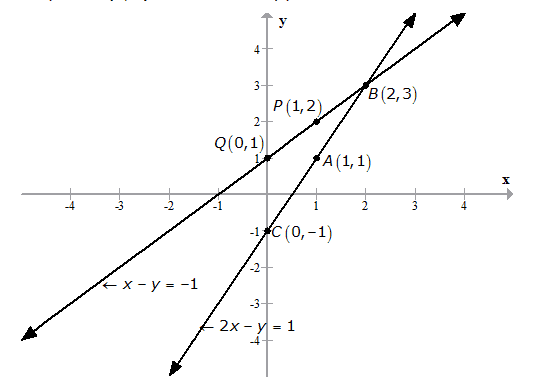The line BQ is the graph of the equation x – y = -1. The two graph lines intersect at point B(2, 3)

Therefore, x = 2, y = 3 is the solution of the given system of equations. The region bounded by these lines and y-axis has been shown by the shaded area.

Question 4: On a graph paper, draw a horizontal line X’OX and a vertical line YoY’ as the x-axis and the y-axis respectively.

2x – 5y + 4 = 0,

2x + y – 8 = 0

Solution:

Given equations are 2x – 5y + 4 = 0 and 2x + y – 8 = 0

Graph of 2x – 5y + 4 = 0:

2x – 5y + 4 = 0 $\Rightarrow y = \frac{2x + 4}{5}$

Putting x = 3, we get y = 2

Putting x = -2, we get y = 0

Putting x = 8, we get y = 4

Hence, the table is,

x3-28y204

Plot the points A(3, 2), B(-2, 0) and C(8, 4) on the graph paper. Join AB and AC to get the graph line BC. Extend it both ways.

Thus, line BC is the graph of 2x – 5y + 4 = 0.

Graph of 2x + y – 8 = 0:

2x + y – 8 = 0 $\Rightarrow y = -2x + 8$

Putting x = 1, we get y = 6

Putting x = 2, we get y = 4

Putting x = 3, we get y = 4

Hence, the table is,

x123y642

Now, on the same graph paper as above plot the points P(1, 6) and Q(2, 4). The point A(3, 2) has already been plotted. Join PA.

Thus, line PA is the graph of 2x + y – 8 = 0. On extending the graph lines on both sides, we find that these graph lines intersect the y-axis at the point R(0, 8) and S(0, 0.8).

Question 5: On a graph paper, draw a horizontal line X’OX and a vertical line YoY’ as the x-axis and the y-axis respectively.

4x – 5y – 20 = 0 and 3x + 5y – 15 = 0

Solution:

Given equations are 4x – 5y – 20 = 0 and 3x + 5y – 15 = 0

Graph of 4x – 5y – 20 = 0:

4x – 5y – 20 = 0 $\Rightarrow y = \frac{4x – 20}{5}$

Putting x = 0, we get y = -4

Putting x = 5, we get y = 0

Putting x = 10, we get y = 4

Hence, the table is:

x0510y-404

Plot the points A(0, -4), B(5, 0) and C(10, 4) on the graph paper. Join AB and BC to get the graph line AC. Extend it both ways.

Thus, line AC is the graph of 4x – 5y – 20 = 0.

Graph of 3x + 5y – 15 = 0:

3x + 5y – 15 = 0 $\Rightarrow y = \frac{-3x + 15}{5}$

Putting x = 5, we get y = 0

Putting x = 0, we get y = 3

Putting x = -5, we get y = 6

Hence, the table is:

x50-5y036

Now, on the same graph paper as above plot the points P(0, 3) and Q(-5, 6). The point B(5, 0) has already been plotted. Join PQ and PB to get the line QB.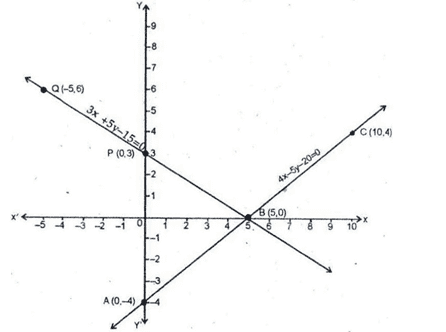Thus, line QB is the graph of 3x + 5y – 15 = 0. The two graph lines intersect at B(5, 0).

Hence, x = 5, y = 0 is the solution of the given system of equations.

Clearly, the vertices of $\Delta PBA$ formed by these lines and the y-axis are A(0, -4), B(5, 0) and P(0, 3).

Question 6: On a graph paper, draw a horizontal line X’OX and a vertical line YoY’ as the x-axis and the y-axis respectively.

4x – 3y + 4 = 0,

4x + 3y – 20 = 0

Solution:

Given equations are 4x – 3y + 4 = 0 and 4x + 3y – 20 = 0

Graph of 4x – 3y + 4 = 0:

4x – 3y + 4 = 0 $\Rightarrow y = \frac{4x + 4}{3}$

Putting x = -1, we get y = 0

Putting x = 2, we get y = 4

Putting x = 5, we get y = 8

Hence, the table is:

x-125y048

Plot the points A(-1, 0), B(2, 4) and C(5, 8) on the graph paper. Join AB and BC to get the graph line AC. Extend it both ways.

Thus, line AC is the graph of 4x – 3y + 4 = 0.

Graph of 4x + 3y – 20 = 0:

4x + 3y – 20 = 0 $\Rightarrow y = \frac{-4x + 20}{3}$

Putting x = 2, we get y = 4

Putting x = -1, we get y = 8

Putting x = 5, we get y = 0

Hence, the table is:

x2-15y480

Now, on the same graph paper as above plot the points P(-1, 8) and Q(5, 0). The point B(2, 4) has already been plotted. Join QB and PB to get the line PQ.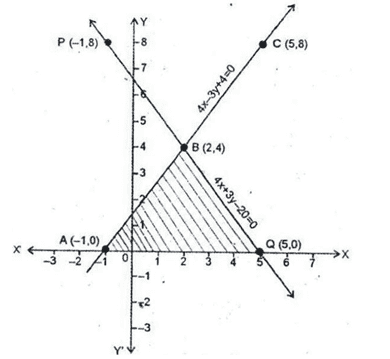Thus, line PQ is the graph of 4x + 3y – 20 = 0. The two graph lines intersect at B(2, 4).

Hence, x = 2, y = 4 is the solution of the given system of equations.

Clearly, the vertices of $\Delta ABQ$ formed by these lines and the x-axis are A(-1, 0), B(2, 4) and Q(5, 0).

Consider the triangle $\Delta ABQ$ :

Height of the triangle = 4 units and base(AQ) = 6 units

Area of the triangle $\Delta ABQ$:

$Area = \frac{1}{2} \times Base \times height = \frac{1}{2} \times 4 \times 6$ sq. units

Area of $\Delta ABQ$ = 12 sq. units

Question 7: On a graph paper, draw a horizontal line X’OX and a vertical line YoY’ as the x-axis and the y-axis respectively.

x – y + 1 = 0, 3x + 2y – 12 = 0

Solution:

Given equations are x – y + 1 = 0 and 3x + 2y – 12 = 0

Graph of x – y + 1 = 0:

x – y + 1 = 0 $\Rightarrow y = x + 1$

Putting x = -1, we get y = 0

Putting x = 1, we get y = 2

Putting x = 2, we get y = 3

Hence, the table is,

x-112y023

Plot the points A(-1, 0), B(1, 2) and C(2, 3) on the graph paper. Join AB and BC to get the graph line AC. Extend it both ways.

Thus, line AC is the graph of x – y + 1 = 0.

Graph of 3x + 2y – 12 = 0:

3x + 2y – 12 = 0 $\Rightarrow y = \frac{-3x + 12}{2}$

Putting x = 0, we get y = 6

Putting x = 2, we get y = 3

Putting x = 4, we get y = 0

Hence, the table is:

x024y630

Now, on the same graph paper as above plot the points P(0, 6) and Q(4, 0). The point C(2, 3) has already been plotted. Join PC and CQ to get the line PQ.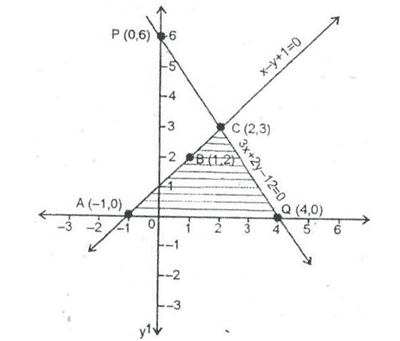Thus, line PQ is the graph of 3x + 2y – 12 = 0. The two graph lines intersect at C(2, 3).

Hence, x = 2, y = 3 is the solution of the given system of equations.

Clearly, the vertices of $\Delta ACQ$ formed by these lines and the x-axis are A(-1, 0), B(2, 3) and Q(4, 0).

Consider the triangle $\Delta ACQ$ :

Height of the triangle = 3 units and base(AQ) = 5 units

Area of the triangle $\Delta ACQ$:

$Area = \frac{1}{2} \times Base \times height = \frac{1}{2} \times 3 \times 5$ sq. units

Area of $\Delta ACQ$ = 7.5 sq. units

Key Features of RS Aggarwal Class 10 Solutions Chapter 3 – Linear Equations In Two Variables – Ex 3B (3.2)

• All the solutions are prepared by subject experts and are accordance to the CBSE guidelines.